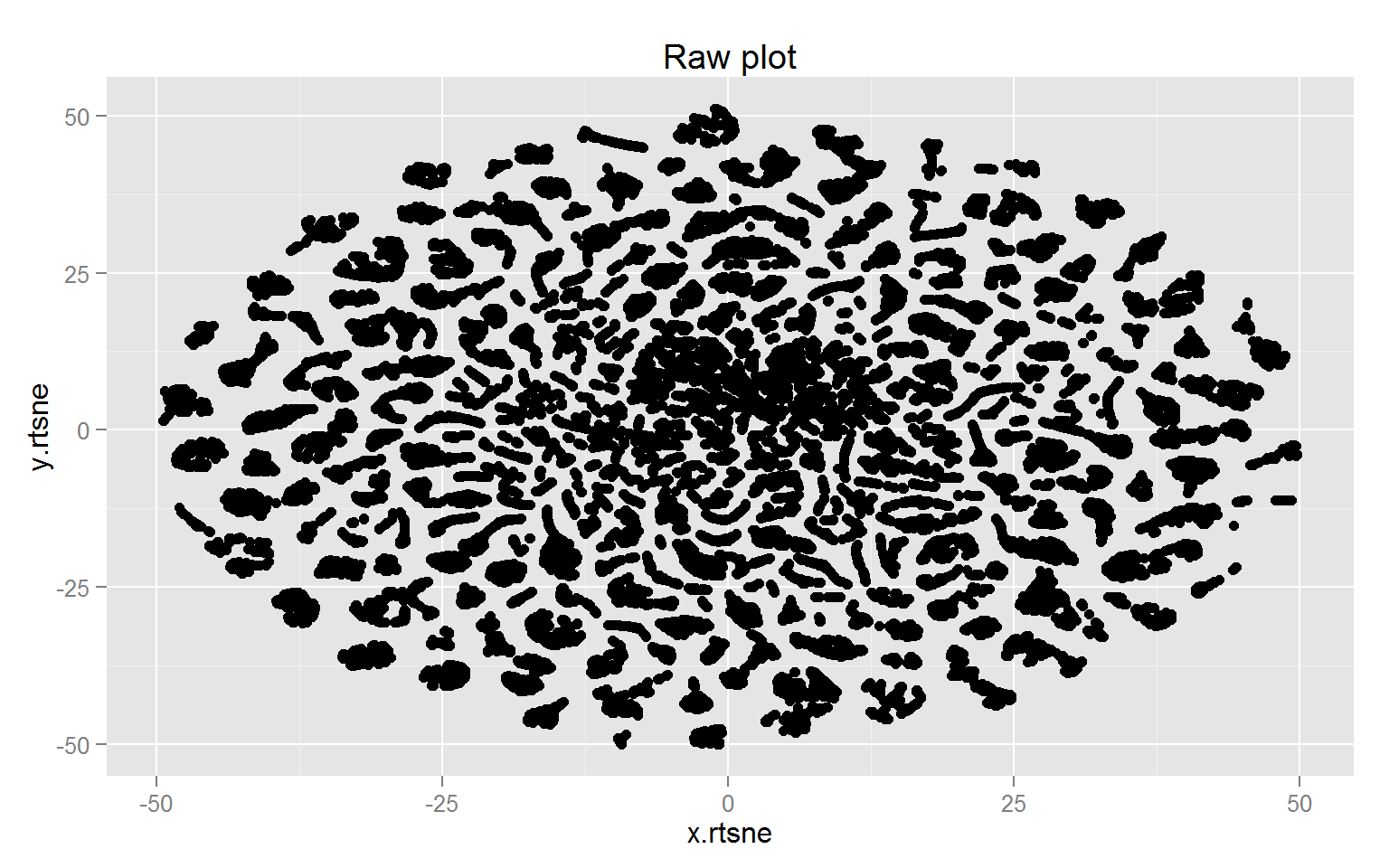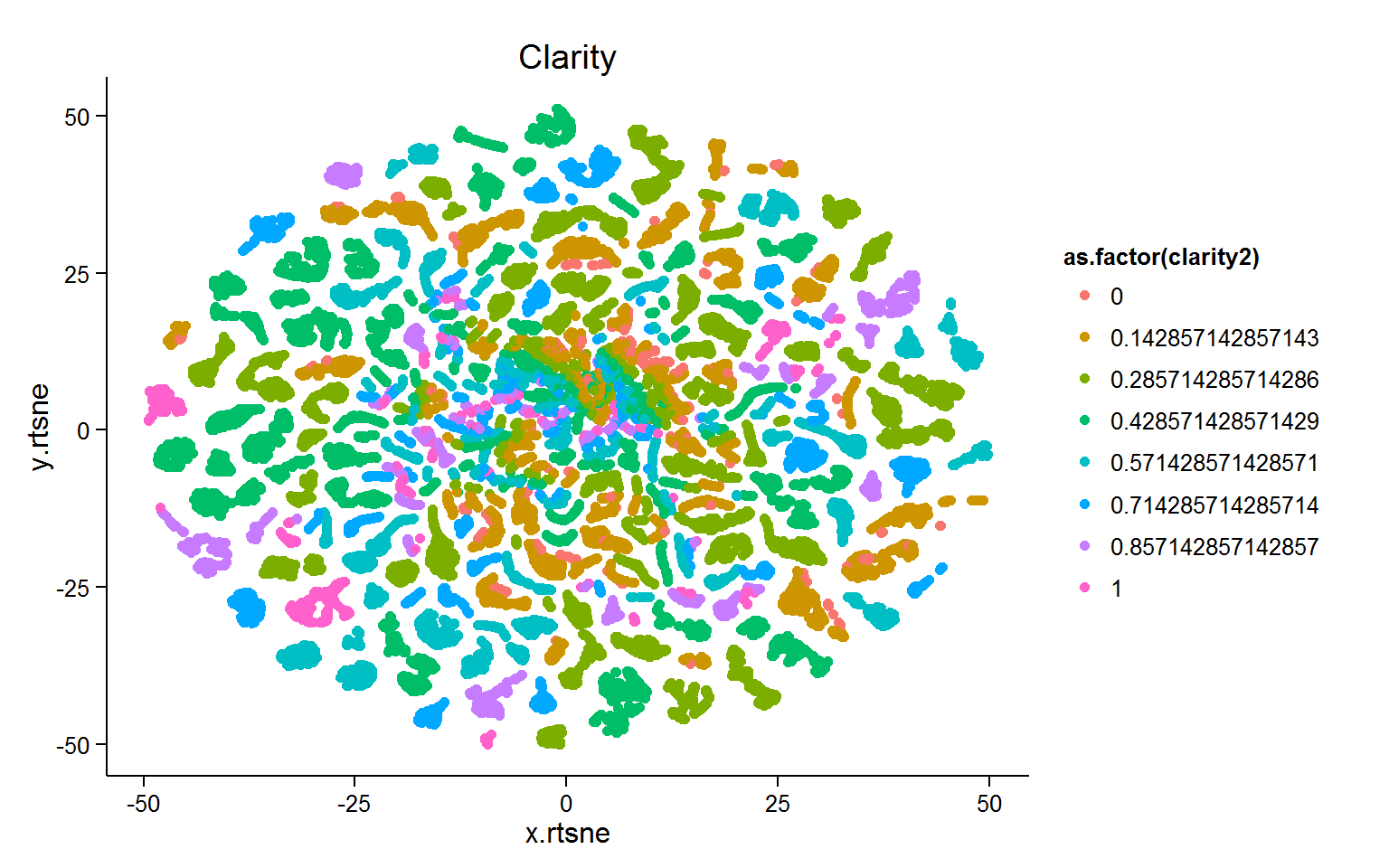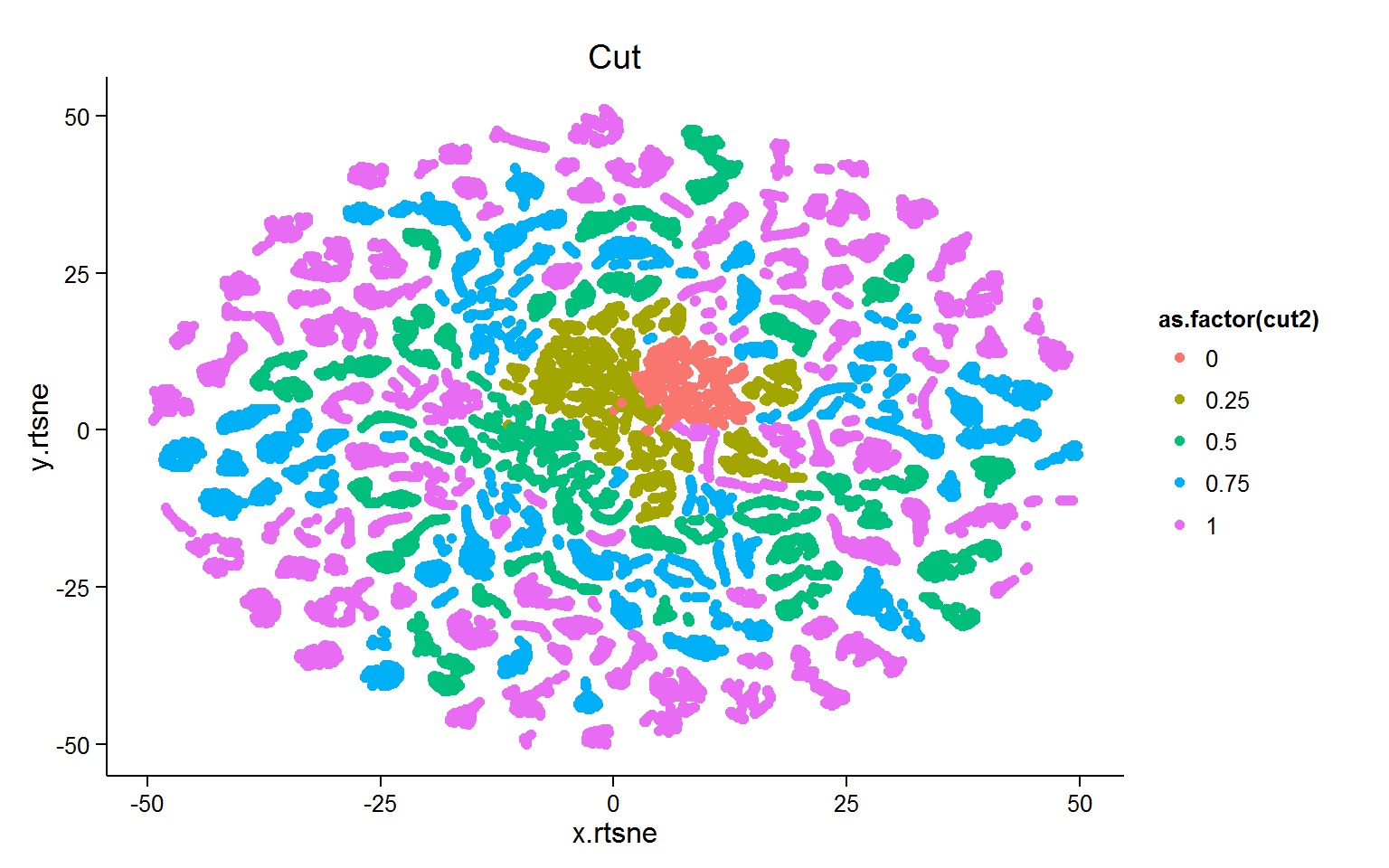# Messing with T-distributed stochastic neighbor embedding

Recently, Kaggle launch the scripts project, which is a board of scripts released by competitioners with possibilites of evaluations by peers.

One of the script is a scatterplot obtained through t-distributed stochastic neighbor embedding which summarise the information of a huge data set.

The scatterplot was so self explaining that I wanted to explore that method.

### Theory

The theory is not that complicated after you catch the idea behind. The original paper is this one.

t-SNE is mainly used to visualise huge data set into scatterplot. It reduces the dimensionality of data to 2 or 3 dimensions, allowing to do 2d or 3d plot.

t-SNE converts distances between datapoint in the original space into conditionnal probabilities $$p_{j|i}$$.

$p_{j|i} = \frac{\exp{(-d(\boldsymbol{x}_i, \boldsymbol{x}_j) / (2 \sigma_i^2)})}{\sum_{i \neq k} \exp{(-d(\boldsymbol{x}_i, \boldsymbol{x}_k) / (2 \sigma_i^2)})}, \quad p_{i|i} = 0,$

$$\sigma_i$$ is the variance of the gaussian which is centered on $$\boldsymbol{x}_i$$. The perplexity parameter of the algorithm can influence this value.

If two points are close, $$p_{j|i}$$ will be high. If two points are far, $$p_{j|i}$$ will be low.

The conditionnal probabilities are used to define the joint probabilities: $p_{ij} = \frac{p_{j|i} + p_{i|j}}{2N}.$

The distances in the embedded space could be describe the same way:

$q_{ij} = \frac{(1 + ||\boldsymbol{y}_i - \boldsymbol{y}_j)||^2)^{-1}}{\sum_{k \neq l} (1 + ||\boldsymbol{y}_k - \boldsymbol{y}_l)||^2)^{-1}},$

The idea now is, for a good visualisation in the embedded space, $$q_{ij}$$ and $$p_{ij}$$ should be equal.

The Kullback-Leibler divergence is the measure used to calculate the mismatch between $$q_{ij}$$ and $$p_{ij}$$.

$KL(P|Q) = \sum_{i \neq j} p_{ij} \log \frac{p_{ij}}{q_{ij}}$

A gradient descent is used to minimise this mismatch.

### The function

The package Rtsne have one function, Rtsne(). To note:

• The function does not allow duplicates
• The SNE result is fairly robust to change in perplexity. Classic values are between 5 and 50
• By defaut, an initial pca is made to reduce the number of dimensions before to do the SNE.
• There is no normalisation made. Consequently, a variable with huge value will appear well separated.
• The algorithm accept only numeric variables.
• Consequently, I personnaly like to divide my dummie variable by the number of modality.
• When I want a specific variable to appear well organised, I increase artificially the value.

### Exemple

Libraries used:

library(data.table)
library(ggplot2) library(Rtsne)

#### Diamond data set

# data table format: diamonds.dt <- data.table(diamonds)
# We transform ordinal variable into numeric one: diamonds.dt[, cut2 := as.numeric(cut)]
diamonds.dt[, clarity2 := as.numeric(clarity)] diamonds.dt[, color2 := as.numeric(color)]
# Normalization of each variable:
diamonds.dt2 <- diamonds.dt[, list(lapply(.SD, function(x) (x - min(x) ) / (max(x) - min(x))), color, cut, clarity), .SDcols = c("carat", "cut2", "color2", "clarity2", "depth", "table", "price", "x", "y", "z")]
# deduplication:
diamonds.dt3 <- diamonds.dt2[, list(count = .N), by = c("carat", "cut2", "color2", "clarity2", "depth", "table", "price", "x", "y", "z", "cut", "color", "clarity")]

diamonds.dt4 <-  diamonds.dt3[, c("carat", "cut2", "color2", "clarity2", "depth", "table", "price", "x", "y", "z"), with = F]
# Embedding the data set:
diamonds.2d <- Rtsne(diamonds.dt4, dims = 2, initial_dims = 50, perplexity = 30, max_iter = 600, verbose = T)
save(diamonds.2d, file = "./DATA/diamonds_2d.rdata")
diamonds.dt3[, x.rtsne := diamonds.2d$Y[, 1]] diamonds.dt3[, y.rtsne := diamonds.2d$Y[, 2]]
# colorless
ggplot(data = diamonds.dt4, aes(x = x.rtsne, y = y.rtsne)) +
geom_point(color = "black") +   ggtitle("Raw plot")# clarity
ggplot(data = diamonds.dt4, aes(x = x.rtsne, y = y.rtsne, color = as.factor(clarity2)))+
geom_point() +   theme_classic() +  ggtitle("Clarity")# cut
ggplot(data = diamonds.dt4, aes(x = x.rtsne, y = y.rtsne, color = as.factor(cut2))) +
geom_point() +   theme_classic() +  ggtitle("Cut")# price
ggplot(data = diamonds.dt4, aes(x = x.rtsne, y = y.rtsne, color = price)) +
geom_point() +   theme_classic() +  ggtitle("Price")